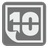Word Pattern
Elementary
English

Sophia's drones are too simple and can recognize symbols in only single words, digits or letters. She wants to teach the drones to understand basic commands which are represented as "words" consisted by letters and digits. For that, Sophia has uploaded "patterns," which describe the sequence of digits and letters in the command. Unfortunately, the drones memory can only store integers and convert them into binary format for comparison. We should help Sophia to write a module for the comparison of patterns and commands.

You are given a pattern as a positive integer and a command as a word. For the comparison, the drone should convert the integer pattern into binary form, append zeros to left for the command length and compare this combination with the command.
1 is a letter. 0 is a digit.
If the pattern and the command are coincided, then return True, else -- False. If pattern is longer than a command, then they are not coincided (For example - 8 = 1000 and "a").

For example. The pattern is 42 and the command is "12a0b3e4".
42 == 101010 in binary form, but this is not enough of length. Let's append zeroes -- "00101010". Then compare:
```      42 == 00101010
12a0b3e4 == DDLDLDLD
--------------------
True    VVVVVVVV```
One more example -- 101 and "ab23b4zz":
```     101 == 01100101
ab23b4zz == LLDDLDLL
--------------------
False    XVXVXXXV```

Input: Two arguments. A pattern as a positive integer and a command as a string.

Output: Is the pattern coincide with the command or not as a boolean.

Example:

```check_command(42, "12a0b3e4") == True
check_command(101, "ab23b4zz") == False
```

How it is used: In this mission you can learn how to store more complex data in simple numbers and how to work with simple patterns. You can expand this concept and use more complex patterns (think about another number systems).

Precondition: 0 ≤ pattern < 231
0 < len(command) < 32
"command" contains only ASCII letters or digits.